# Tax Evasion Cube Spiral

Group Members

Sam Janssen (Designer)
Basilios Dallas (Math Consultant)
Michael Mathis (Writer)

First Design Iteration

We opted to make a cube-based spiral fractal.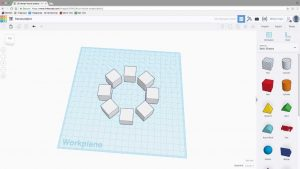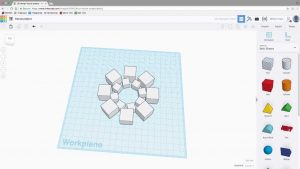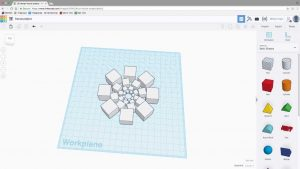Originally, we were thinking about doing a chain fractal based off of doughnut-shaped tubes, but we got inspired by the spiral cube fractal made in class. So once we had found a preset object that included a literal ring of cubes, we were onboard immediately with trying out our own iteration. In our iteration, each cube in each descending ring is about 3/5 the size smaller of the previous cube in the previous ring. For example, the outermost cube is about 60mm, and the next outermost is about 36mm. In addition, the ring of cubes moves about 15 degrees clockwise. Design-wise, think of it as a reverse to the Pythagoras Tree fractal. We were able to design one with 3 visible levels/layers. It was relatively easy to design (thank god there was a preset), but still a very pleasing image.

The design might be difficult to print due to the density of all the cubes. Creating a Menger Sponge is already an hours worthy project, so, unless the lack of holes makes the printing of cubes shorter, we can expect this to be a long process. In addition, we wonder if the smaller cube rings will turn out as good as the bigger rings, because previous smaller prints have been brittle, incomplete, and unpleasing to view. Nonetheless, we are very excited to attempt this fractal and look forward to improving it any way we can!

First Print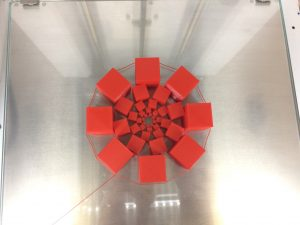To begin, our very first full-size print looked pretty good. Structurally, it was exactly what our design intended. Unfortunately, the whole thing fell apart after we took it off. Disappointing, don’t we know.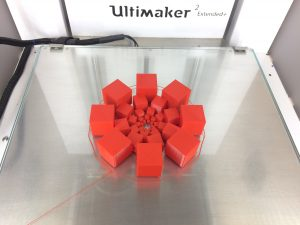However, we did a second print. After adjusting some of the dimensions, and creating a plate to hold together the foundation, our new model is much stronger and durable. We are obviously careful, but it will not fall apart like the last one.

### Volume – take the outermost group of cubes, and you calculate the volume. In our final print, each individual cube in the outer-layer had a volume of about 1cm^3. On the next level, the cubes shrink to 60% or 3/5 size of the previous level. This continues to happen infinitely.

So, on the first level, the volume of one cube is 1 cm^3, and thus the volume for level 1, and the total volume for the whole shape would equal 8 cm^3.

So we calculated the total volume with each corresponding level from there:

The second level, [1 + (.63)]*8 =9.728 cm3

The third level is [1 + (.63) + (.63)2]*8= 10.1012 cm3

The fourth level is [1 + (.63) +(.63)2 +(.63)3]*8 = 10.1992 cm3

The fifth level is [1 +(.63(.63)2 +(.63)+(.63)4]*8 =10.1993 cm^3

The sixth level is [1 + (.63) + (.63)2 + (.63)3+ (.63)+ (.63)5]*8 =10.2030 cm3

(You can replace the .6 with 3/5 of the previous level, whatever the volume of the first cube)

When you finish calculating the volume for one cube on each level, you can now multiply each level by 8, or once you add up the volume of 1 cube from each level, you just multiply that number by 8, because the ring contains 8 cubes.

This fractal will continue to do this forever, and will continue to approach zero but will not ever reach it because each level is 3/5 or .6 of the previous level, so there will always be a smaller cube. We can’t quite make it this way, so we lose some volume in our print because we have to make the cubes connect in order for us to have a model. However, in an ideal world, if this were to go infinitely, it would never reach a volume beyond 20cm3. Also, in an ideal world, every cube is rotated 15° so that it will just barely touch the previous level.

### Dimension Calculations

For our dimension calculations, we decided to solve for the dimensions of a Sierpinski Carpet. The Sierpinski Carpet is a plane fractal first designed by Waclaw Sierpinski in 1916. It is a relatively simple fractal to calculate, but how do we calculate its dimensions. What do we need to find?

Sd=N

Let’s break down each variable:

S is equal to the scale-down factor. This is the scale to which the smaller pieces of a fractal compare to the larger ones. From our calculations, we know that every piece is about 1/3 of its previous iteration.

So, S=3.

Next, N is equal to the number of copies. See, every time the fractal begins a new iteration, the fractal copies itself into a certain amount of divisible sections. With the Sierpinski Carpet, we know that the fractal has 8 distinct sections, of which it creates new copies of itself for with each new level.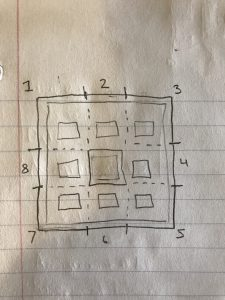The Sierpinski Carpet can be broken up into 8 divisible parts. These are all scaled-down copies of the original Sierpinski Carpet.

So, n = 8.

Our final equation:

3d=8

That just leaves d, or the dimension we are solving for. So let’s solve:

3d=8

(d)log3 = log8

d = log8/log3

d (or dimension) = 1.8928